# Restrict Dates using Data Validation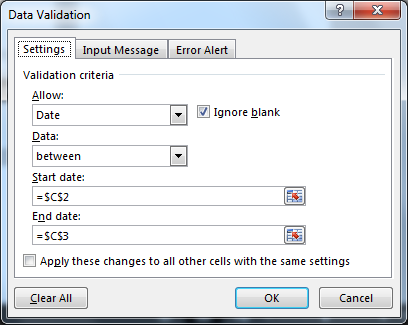In this article, we will learn How to validate date entries which contain specific year, specific month or specific date using data validation in excel.

Data validation

Data validation tool restricts the user to input data to ensure its accuracy and consistency in Excel. It helps in the consistency of the data. It guides the user to input the type of option which is necessary for your worksheet. Validation of text entries which contain specific substring is done with the use of Excel formula. Following steps will be a guide, how to validate text entries having specific criteria.

Sometimes we want users to pick dates from specific dates only. Irregular dates makes it hard to analyse data effectively and increase work overhead. In this article, we will learn how to put data validation for specific date only and make users enter dates only from that time interval.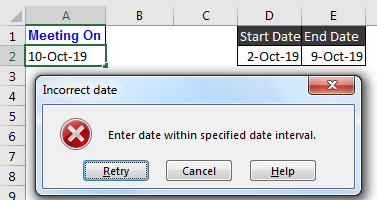How to Restrict Dates using Data Validation in Excel?

If you want to prevent user from entering dates of their choice in specific cells, then this article is for you. In this article we will learn how to restrict user to enter the dates which are preset by the owner of this file.

The function used in this tutorial will work on following versions of Microsoft Excel:

Excel 2013, Excel 2010, Excel 2007, Excel 2003

Let’s assume the Start Date is 1/1/2014 & End Date is 12/31/2014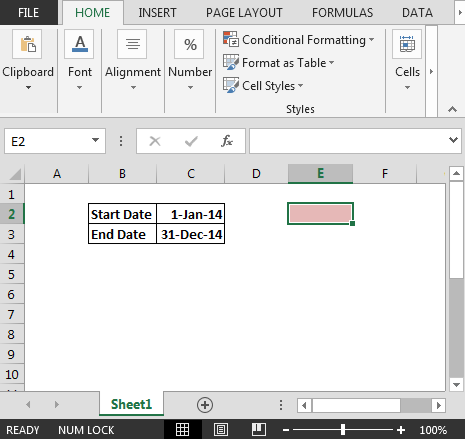To restrict user from entering date beyond the specified dates, we need to follow the below steps:

• Select the cells in which data validation needs to be applied.
• Press ALT + D + L
• From Settings tab, select Date in Allow dropdown
• In Start date, enter cell C2 (Start Date)
• In End date, enter cell C3 (End Date)
•• As we click on OK button; the user will not be allowed to enter any date apart from the specified dates.

In this way, we can prevent user from entering any date of their choice.

#### Example: Put Date Validation in Excel

Here I have an specified date interval in cell D2 and E2. In D2, start date is 2-Oct-19 and in E2, end date is 9-Oct-19. In cell A2 user will enter a meeting date. The criteria is that date can not be out of the above specified time interval.

To put date validation in Excel, follow these steps:

1. Select cell/s, where you want to put validation.
2. Go to Data->Validation. You can use keyboard shortcut ALT>A>V>V.
3. In allow, select custom.
4. In Formula., write this formula  =AND(A2>=\$D\$2,A2<=\$E\$2)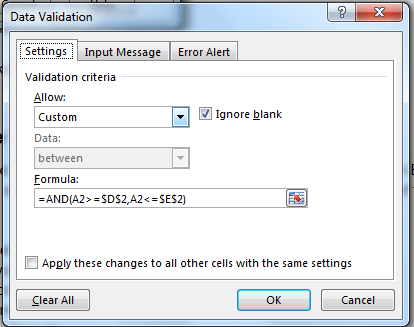5. In error alert, write this line in Error message box. “Enter date within specified date interval.”
6. Hit Ok. The work is done.

Now try to write any date which is before or after the given date in D2 and E2. Excel will not accept the input.

As you change the dates in start date and end date, the validation will change. There will be no need to change data validation.

But if you want to hardcode the dates then write this formula in formula input box of data validation.

 =AND(A2>=DATE(2019,10,2),A2<=DATE(2019,10,2))

This will make the start date and end date fixed. You can copy A2 and paste special for data validation only to apply on other cells.

How it works?

Well, here we are using AND function to check if user is entering the correct data.AND(A2>=\$D\$2,A2<=\$E\$2): Here, excel first checks the first criteria. If value in A2 is greater than or equal to value in D2, it return TRUE else FALSE.Next excel checks the second criteria that if value in A2 is less than or equal to value in E2. If it is, than it returns TRUE else FALSE.

If either of the criteria returns FALSE, input is rejected.

Here are all the observational notes regarding using the formula.Notes:

1. Apply the data validation tool to restrict the user to input invalid value which doesn't satisfy the condition.
2. Input date value must be in valid date format or else the cell doesn't accept the input value.
3. Formula inside the data validation tool can be customized.
4. YEAR, MONTH & DAY function extracts the year, month or day value from the valid input date value.
5. Operators like equals to ( = ), less than equal to ( <= ), greater than ( > ) or not equals to ( <> ) can be performed within function applied with numbers only.

Hope this article about how to do Data validation with specific year, month, day in excel is explanatory. Find more articles on Date & Time functions here. If you liked our blogs, share it with your friends on Facebook. And also you can follow us on Twitter and Facebook. We would love to hear from you, do let us know how we can improve, complement or innovate our work and make it better for you. Write us at info@exceltip.com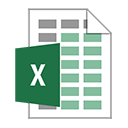Related Articles:

Data Validation in Excel : restricts user to input value manually using this tool in Excel.

Way to use Vlookup function in Data Validation : allow values from the vlookup table in Excel.

Restrict Dates using Data Validation : allow dates in the cell which lays within Excel date format.

How to give the error messages in Data Validation : customize your data validation cell with error messages.

Create Drop Down Lists in Excel using Data Validation : restricts users to input data manually.

Popular Articles:

50 Excel Shortcut to Increase Your Productivity : Get faster at your task. These 50 shortcuts will make you work even faster on Excel.

How to use the VLOOKUP Function in Excel : This is one of the most used and popular functions of excel that is used to lookup value from different ranges and sheets.

How to use the COUNTIF function in Excel : Count values with conditions using this amazing function. You don't need to filter your data to count specific values. Countif function is essential to prepare your dashboard.

How to Use SUMIF Function in Excel : This is another dashboard essential function. This helps you sum up values on specific conditions.

1.